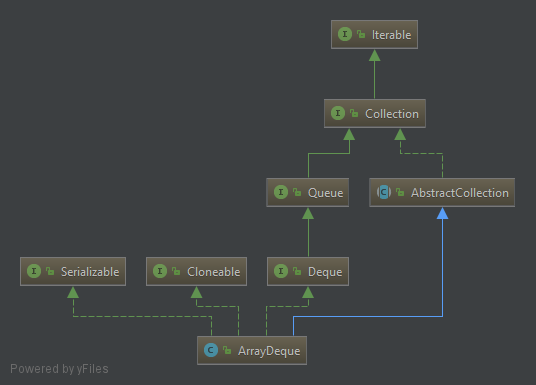Tags: Java ArrayDeque

In this tutorial we will learn how to use the java.util.ArrayDeque class, a member class of Java Collections Framework. We will go through different examples to see how this class suppport methods that allow you to insert and retrieves element in both front and end of the element list. ArrayDeque is an implementation of Deque interface with class diagram as below:#### How to use ArrayDeque as a stack

We can push an element onto the stack and pop it from stack using ArrayDeque as below

``````Deque<Integer> deque = new ArrayDeque<>();
deque.push(1);
deque.push(2);
deque.push(3);
deque.push(4);
Integer element = deque.pop();``````

#### How to use ArrayDeque as a queue

We can use offer and poll method to populate elements in queue as below

``````Deque<Integer> queue = new ArrayDeque<>();
queue.offer(1);
queue.offer(2);
queue.offer(3);
queue.offer(4);
Integer element = queue.poll();``````

#### Different method to iterate over elements of the deque

The class provide two methods to return an iterator:

• descendingIterator method to return an iterator over the elements in reverse sequential order.
• iterator method to return an iterator over the elements.
``````Deque<Integer> stack = new ArrayDeque<>();
stack.push(1);
stack.push(2);
stack.push(3);
stack.push(4);

Iterator descendingIterator = stack.descendingIterator();
while(descendingIterator.hasNext()) {
System.out.println(descendingIterator.next());
}

Iterator iterator = stack.iterator();
while(iterator.hasNext()) {
System.out.println(iterator.next());
}``````

Beside that, there are some other helpful methods when you using ArrayDeque:

• size to get number of elements.
• toArray to return an array of all elements.
• isEmpty to check whether the ArrayDeque is empty or not.
• contains to check a specified element be contained in ArrayDeque or not.
``````Deque<Integer> stack = new ArrayDeque<>();
stack.push(1);
stack.push(2);
stack.push(3);
stack.push(4);

System.out.println(stack.size());

Object[] objectArray = stack.toArray();

Integer[] integerArray = stack.toArray(new Integer);

System.out.println(stack.isEmpty());

System.out.println(stack.contains(2));
System.out.println(stack.contains(5));``````

That’s all for a simple examples how to use java.util.ArrayDeque class.